Newton’s laws of motionPage 6

WATCH ALL SLIDES

Slide 44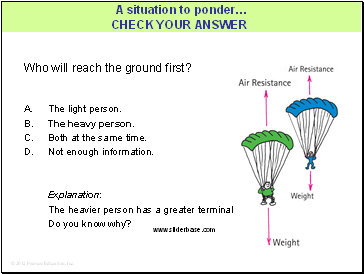Who will reach the ground first?

A. The light person.

The heavy person.

Both at the same time.

Not enough information.

Explanation:

The heavier person has a greater terminal velocity.

Do you know why?

A situation to ponder…

Slide 45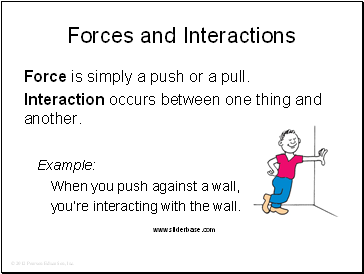Forces and Interactions

Force is simply a push or a pull.

Interaction occurs between one thing and another.

Example:

When you push against a wall,

you’re interacting with the wall.

Slide 46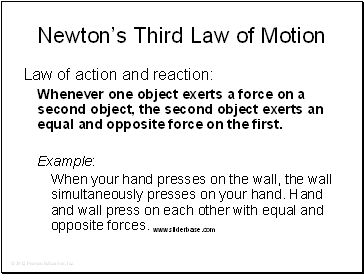Newton’s Third Law of Motion

Law of action and reaction:

Whenever one object exerts a force on a second object, the second object exerts an equal and opposite force on the first.

Example:

When your hand presses on the wall, the wall simultaneously presses on your hand. Hand and wall press on each other with equal and opposite forces.

Slide 47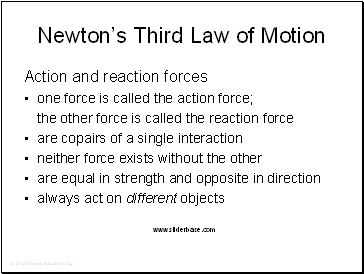Newton’s Third Law of Motion

Action and reaction forces

one force is called the action force;

the other force is called the reaction force

are copairs of a single interaction

neither force exists without the other

are equal in strength and opposite in direction

always act on different objects

Slide 48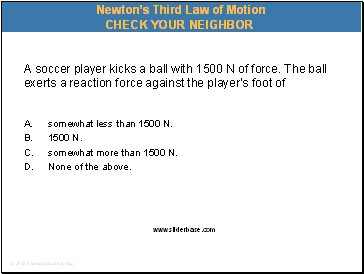A soccer player kicks a ball with 1500 N of force. The ball exerts a reaction force against the player’s foot of

A. somewhat less than 1500 N.

1500 N.

somewhat more than 1500 N.

None of the above.

Newton’s Third Law of Motion

Slide 49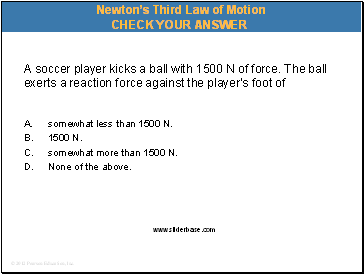A soccer player kicks a ball with 1500 N of force. The ball exerts a reaction force against the player’s foot of

A. somewhat less than 1500 N.

1500 N.

somewhat more than 1500 N.

None of the above.

Newton’s Third Law of Motion

Slide 50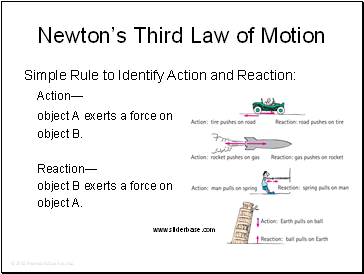Newton’s Third Law of Motion

Simple Rule to Identify Action and Reaction:

Action—

object A exerts a force on

object B.

Reaction—

object B exerts a force on

object A.

Slide 51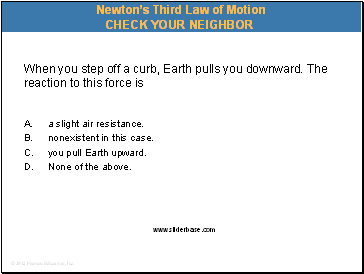When you step off a curb, Earth pulls you downward. The reaction to this force is

A. a slight air resistance.

nonexistent in this case.

you pull Earth upward.

None of the above.

Newton’s Third Law of Motion